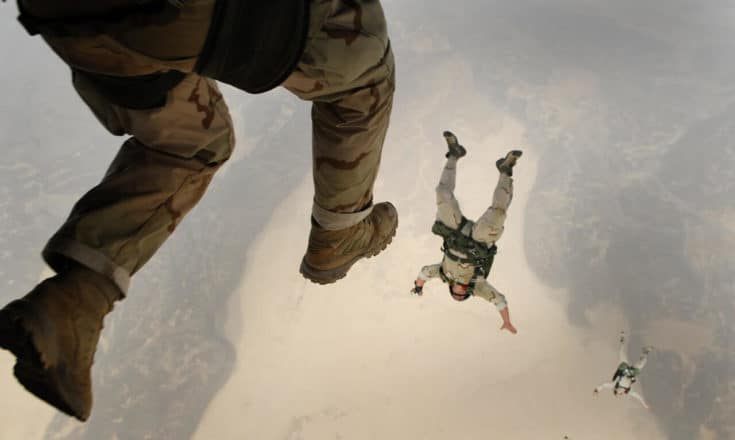PhysicsTeacher.in

High School Physics BLOG - online class notesRSS Feed

vertical motion under gravityNumerical problems on Vertical motion

In this post we will solve harder numerical problems on Vertical motion physics. vertical motion is an interesting chapter in mechanics . Here you get selected problems to solve which will help you to test your understanding.Solution to problems – class 9 – Set 1 Q 48

Problem Statement 48) A ball is thrown vertically upwards from the top of a tower with an initial velocity of 19·6 m/s. The ball reaches the ground after 5 s. Calculate: (i) the height of the tower, (ii) the velocity of ball on reaching the ground. Take g = 9·8 m/s^2 Follow my blog withSolution to problems – class 9 – Set 1 Q 30

Problem Statement A baseball is popped straight up into the air and has a hang-time of 6 s. Determine the height to which the ball rises before it reaches its peak. (Hint: the time to rise to the max height is one-half the total hang-time.) Solution Hang time = Total time required for upward plusPhysics problems for class 9 – numericals with solutions-set1 (CBSE / ICSE)

101 physics Numerical problems with solution – appropriate for high school (class / grade 9 , 10, 11, 12), IIT foundation, other exams including competitive examinations. An ever growing problem set.

Older Posts››
error: Content is protected !!